Using this action, you can perform arithmetic operations on two numbers or numeric variables.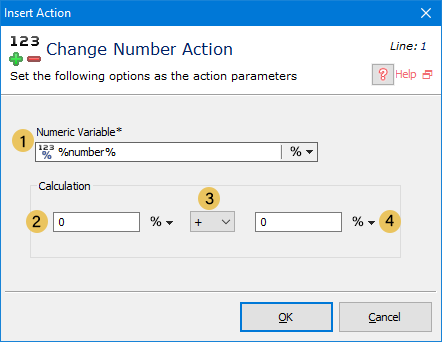Description of the parameters: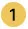Numeric Variable: The result of the arithmetic operations will be placed in this variable. You can use the % sign to select the variable that you want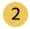First Number: You can insert a number or use the % sign to select the variable that you want.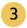Operator: Can be one of the following

• ( +
• _
• *
• /
• MOD (the remainder of the division of two numbers)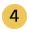Second Number: You can insert a number or use the % sign to select the variable that you want.5 From 147

#### Web Scraping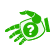vUser website support You can ask your questions